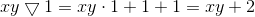SSAT Upper Level Math : How to find algebraic patterns

Example Questions

1 3 Next →

Example Question #21 : Patterns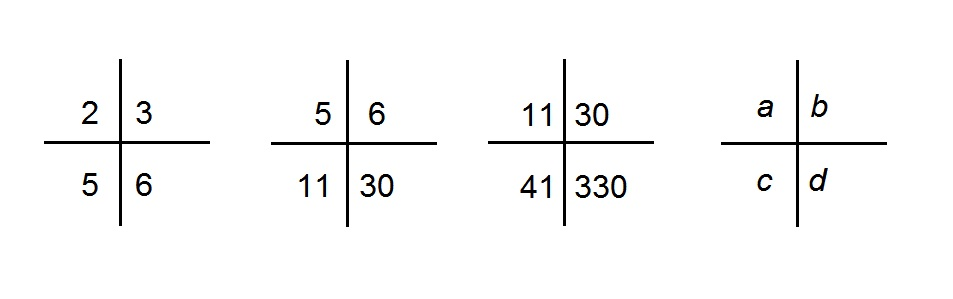The above diagram shows a sequence of figures. In the fourth figure, each of the four variables,, and, is replaced by a value.

What value replaces?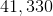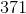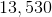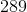Explanation:

In each of the crosses, the lower left and lower right entries are the sum and product of the top two entries, respectively. The top two numbers of the next cross are the same as the bottom two of the current cross. Therefore, the fourth figure has as its top two entries 41 and 330 (the bottom two of the third figure).is equal to their sum,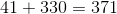.

Example Question #22 : Patterns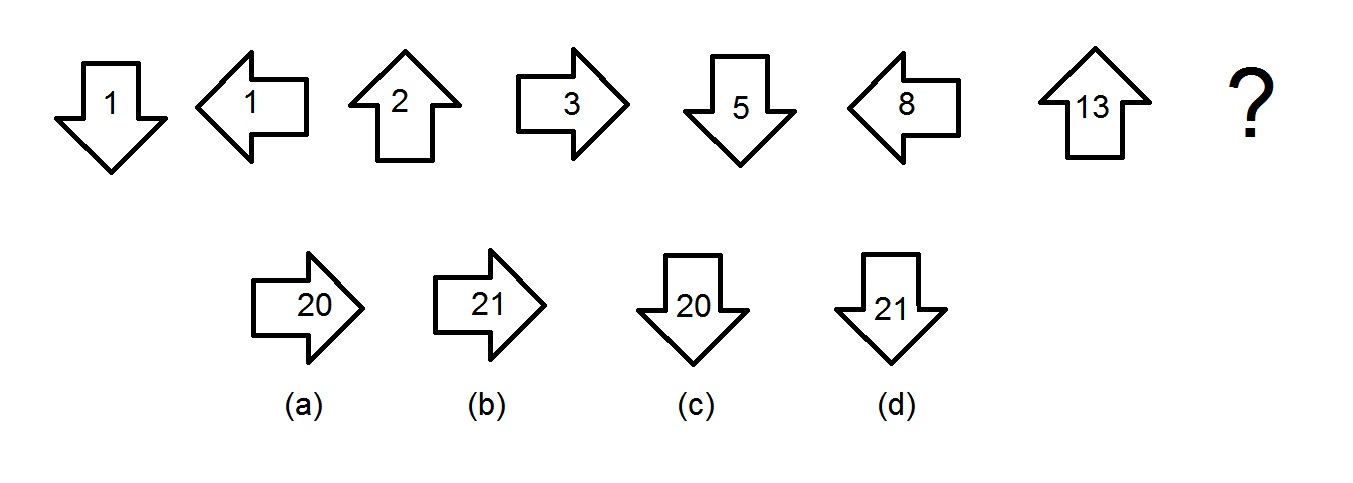The top row in the above diagram shows a sequence of figures. Which figure in the bottom row is the next one in the sequence?

Figure (b)

Figure (a)

Figure (d)

Figure (c)

Figure (b)

Explanation:

Going from figure to figure, the arrow is rotating one quarter of a turn clockwise each time. Therefore, in the next figure, the arrow should be pointing to the right, thereby eliminating Figures (c) and (d) as choices and leaving Figures (a) and (b).

Starting with the third figure, each number is obtained by adding the numbers in the previous two figures (this is called the Fibonacci sequence), as follows: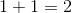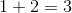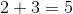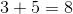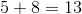The number inside the next arrow is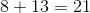so the correct choice is Figure (b).

Example Question #23 : PatternsThe above diagram shows a sequence of figures. In the fourth figure, each of the four variables,, and, is replaced by a value.

What value replaces?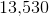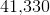Explanation:

In each of the crosses, the lower left and lower right entries are the sum and product of the top two entries, respectively. The top two numbers of the next cross are the same as the bottom two of the current cross. Therefore, the fourth figure has as its top two entries 41 and 330 (the bottom two of the third figure).is equal to their product,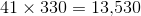.

Example Question #24 : Patterns

A sequence of numbers begins: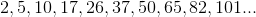What is the one-hundredth entry in this sequence?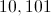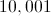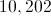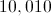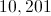Explanation:

Let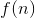be the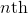entry in the sequence. Then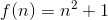. The one-hundredth entry is therefore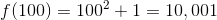Example Question #25 : Patterns

Define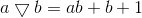Which of the following expressions is equal to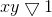?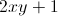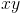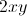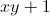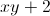Replacewith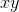andwith 1: